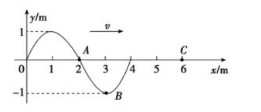$s$ 时质点 $A$ 刚好第 3 次到达波峰. 下列说法正确的是 . (填正确答案标号. 选对 1 个得 2 分, 选对 2 个得 4 分, 选对 3 个得 5 分. 毎选错 1 个扣 3 分, 最低得分为 0 分)

A. 该波的波速一定为 $1 \mathrm{~m} / \mathrm{s}$
B. 如果该波在传播过程中与频率为 $0.5 \mathrm{~Hz}$ 的横波相遇, 一定发生干涉现象
C. 质点 $C$ 起振方向沿 $y$ 轴负向
D. 如果质点 $C$ 到达波峰, 则质点 $B$-定在平衡位置
E. 质点 $A$ 的振动方程可表示为 $y=\sin (0.25 \pi t) \mathrm{m}$ACD